# Tag: functions## Hangman in python with source code

Introduction Hangman is a simple pencil and paper guessing game. Two or more players can play this game at once. The players have to guess the unknown word.## Hirst painting in python

Introduction A hirst painting is a random painting made with hirst. It generally creates dot patterns with different colors. We use python turtles library.## Presentation layer

Introduction The presentation layer is the layer 6 of the OSI reference model. It is responsible for defining how information is transmitted and presented to the user interface.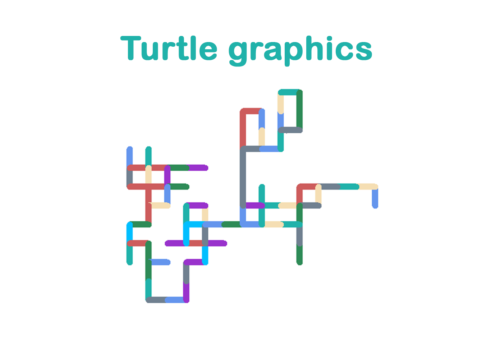## Turtle graphics

Introduction Turtle graphics is the graphical user interface python libraries. Turtle graphics is an awesome and simple way of introducing programming to the kids.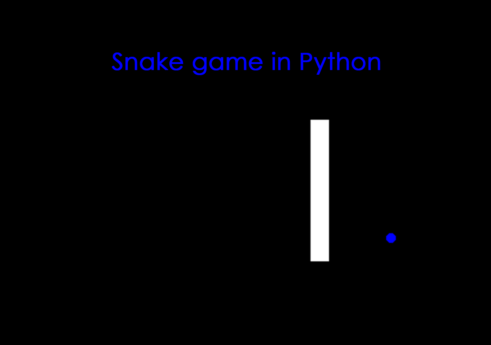## Snake game project in python with source code

Introduction Snake game project is a fun game based project made using turtle graphics library. This is a regular snake game that search foods and grows longer. Turtle is an inbuilt module in python that …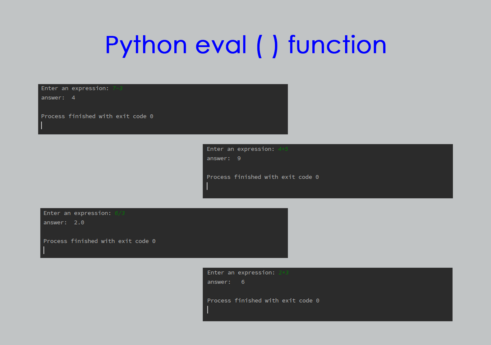## python eval() function

Introduction Python eval is a built in python function useful for calculations. This function evaluates in the process of finding the output.## Average height in python with source code

Introduction Average height is a programming exercise in python. This program finds the average height from various numbers of heights entered.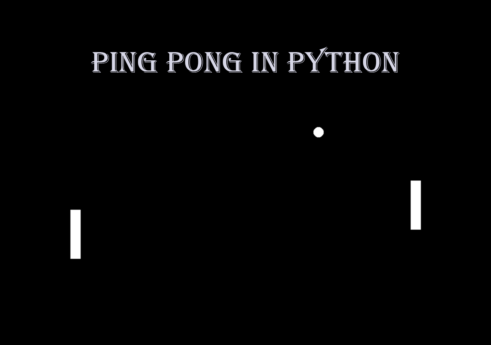## Ping pong in python with source code

Introduction Ping pong is a game based python project made using turtle library. It is the famous ping pong multiplayer game played between two players.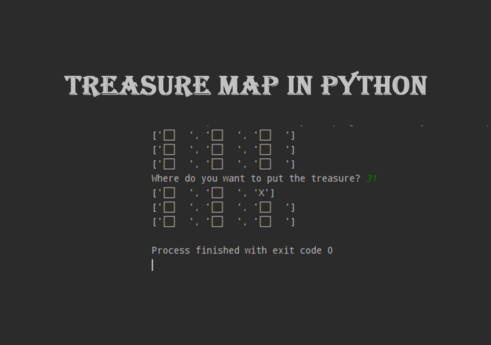## Treasure map in python with source code

Introduction Treasure map is a python based programming project. The use of this project is marking the mark on the map. It is easy to use and implement.## Highest score in python with source code

Introduction Highest score is a python based programming project. It is the best way of finding the largest score among multiple scores.